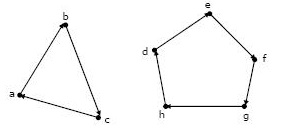Aptitude | GATE CS 1998 | Question 66

• Last Updated : 12 Oct, 2017

a.  Prove by induction that the expression for the number of diagonals in a polygon of n sides is

n(n-3) /2.

b.  Let R be a binary relation on A = {a, b, c, d, e, f, g, h} represented by following two component digraph. Find the smallest integers m and n such that m<n and  R= Rn